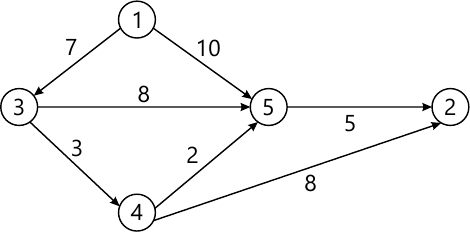MORE IN Analysis of Algorithms
MU Computer Engineering (Semester 4)
Analysis of Algorithms
December 2015
Total marks: --
Total time: --
INSTRUCTIONS
(1) Assume appropriate data and state your reasons
(2) Marks are given to the right of every question
(3) Draw neat diagrams wherever necessary

1 (a) Define 0, Ω, and θ notations. To find the complexity of given recurrence relation.
i) T(n)=4T(n/2)+n3
ii) T(n)=2T (n/2)+n3.
10 M
1 (b) Implement the binary search, and derive its complexity.
10 M

2 (a) Explain 0/1 knapsack problem using dynamic programming.
10 M
2 (b) Explain optimal storage on tapes and find the optimal order for given instance.
n=3, and (11, 12, 13)=(5, 10, 3).
10 M

3 (a) Let n=4, (p1, p2, p3, p4)=(100, 10, 15, 27) and (d1, d2, d3, d4) = (2, 1, 2, 1). Find feasible solutions, using job sequencing with deadlines.
10 M
3 (b) Find a minimum cost path from 3 to 3 in the given graph using dynamic programming.10 M

4 (a) Explain 8 Queen problem.
10 M
4 (b) Explain sum of subset problem. Find all possible subsets of weight that sum to m, let n=6, m=30, and w[1:6]={5, 10, 12, 13, 15, 18}
10 M

5 (a) Write an algorithm for Kunth-Morrie-Pratt (KMP).
10 M
5 (b) Explain the Strassen's Matrix multiplication.
10 M

Write note on (any two):
6 (a) Randomized Algorithms.
10 M
6 (b) Branch and bound strategy
10 M
6 (c) Huffman coding.
10 M
6 (d) Rabin karp algorithm
10 M

More question papers from Analysis of Algorithms